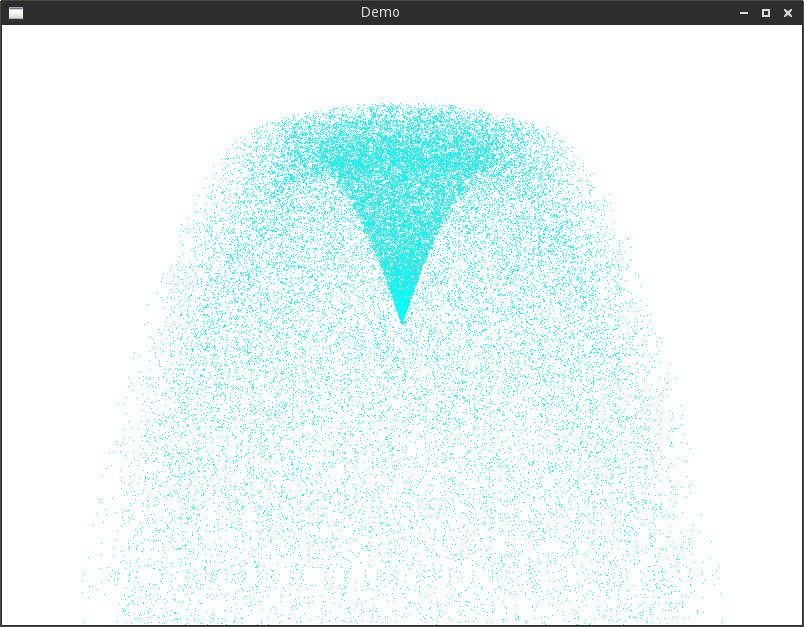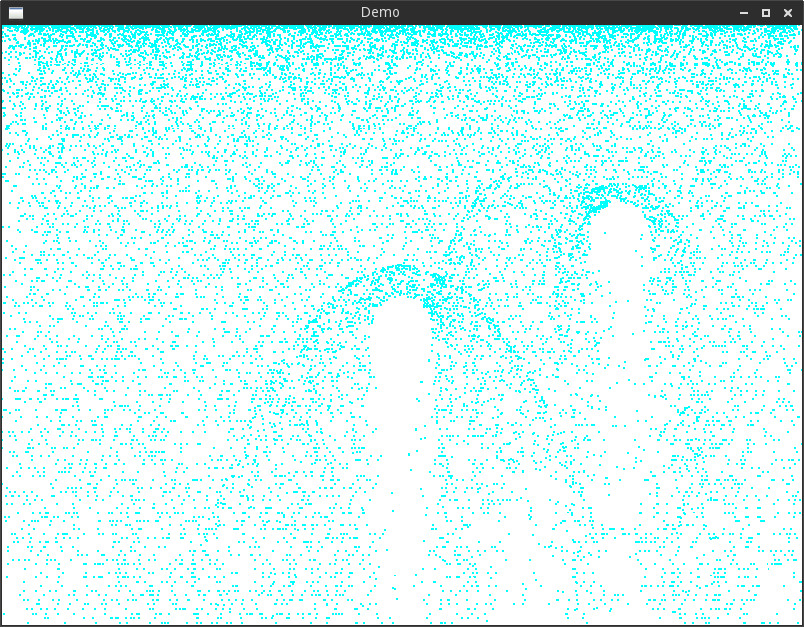# Introduction

Grain is a domain specific language (DSL) to program GPU-accelerated particle systems. This is an academic project for CS2104R (Programming language concepts).

It consist of 2 parts:

• `grainc`: a compiler which transforms grain code into GLSL
• `grainr`: a runtime library to use the output of `grainc`

Please refer to the project report for more details such as motivations and syntax.

# My role and contributions

This is an individual assignment and I am the only developer of this project. My contributions include:

# Technologies and tools used

• C/C++ for both the compiler and the runtime API
• GLSL as the compilation target
• OpenGL 3.0
• Open source gsls-optimizer library for syntax verification and optimization of generated code
• CMake as the build tool

# Samples

The following piece of code creates a point emitter:

``````@param vec2 emission_point
@param float min_speed
@param float max_speed
@param float min_angle
@param float max_angle
@attribute vec2 position
@attribute vec2 velocity
@require aging

position = emission_point;
float speed = random_range(min_speed, max_speed);
float angle = random_range(min_angle, max_angle);
velocity = vec2(cos(angle), sin(angle)) * speed;
``````

Combined with a particle system that reacts to gravity, it creates the following effect:The following code creates an object which deflects particles:

``````@require linear_motion
@param vec2 center

vec2 normal = position - center;
bool inCircle = length(normal) < radius;
bool goingIn = dot(normal, velocity) < 0.0;
bool bounce = inCircle && goingIn;
vec2 newV = reflect(velocity, normalize(normal));
velocity = (bounce) ? newV / 3 : velocity;
life = select(bounce, life / 2, life);
``````

Here’s a screenshot of it in action:# Source code

Available at: https://github.com/bullno1/grain